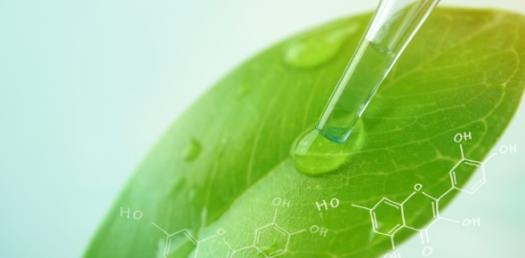# Physical Science - Ch. 1 - The Nature Of Science

10 Questions | Attempts: 773SettingsCh. 1 Key Vocabulary and Concepts - Choose the best answer for each question. Be sure to read all of the answers before choosing. God bless you!

• 1.
When designing an experiment, the first step is to ___________.
• A.

State a hypothesis

• B.

Draw conclusions

• C.

List a procedure

• D.

Analyze the data

• E.

State the problem

• 2.
A factor that does not change in an experiment is the ___________.
• A.

Control

• B.

Hypothesis

• C.

Data

• D.

Constant

• E.

Independent variable

• 3.
The reason for wearing safety goggles in the laboratory is to ______________.
• A.

Improve eyesight.

• B.

Protect the eyes from harmful substances

• C.

Protect the clothes from chemical spills

• D.

Keep your clothes away from the fire

• E.

Apply the procedure

• 4.
An organized process used to gather observations and test a hypothesis is _________.
• A.

A problem

• B.

An experiment

• C.

An experience

• D.

An exercise

• E.

A constant

• 5.
The lightbulb is an example of ____.
• A.

Pure science

• B.

Technology

• C.

A dependent variable

• D.

An exercise

• E.

A hypothesis

• 6.
An idea, structure, or system that can be used to represent something you are trying to explain is a ____________.
• A.

Model

• B.

Constant

• C.

Control

• D.

Hypothesis

• E.

Variable

• 7.
A physical scientist who studies heat and light is working with _______.
• A.

Properties of matter

• B.

Energy

• C.

Living organisms

• D.

Matter

• E.

Dependent variables

• 8.
Physical science is the study of _________.
• A.

Diverse living things

• B.

Biomes

• C.

Matter

• D.

Energy

• E.

Matter and energy

• 9.
A rule or principle that describes the behavior of something in nature is a ______________.
• A.

Hypothesis

• B.

Conclusion

• C.

Theory

• D.

Scientific law

• E.

Variable

• 10.
The application of science knowledge to practical problems is __________________.
• A.

Pure science

• B.

Hypothesis

• C.

Discovery

• D.

Control

• E.

Technology

## Related TopicsBack to top
×

Wait!
Here's an interesting quiz for you.